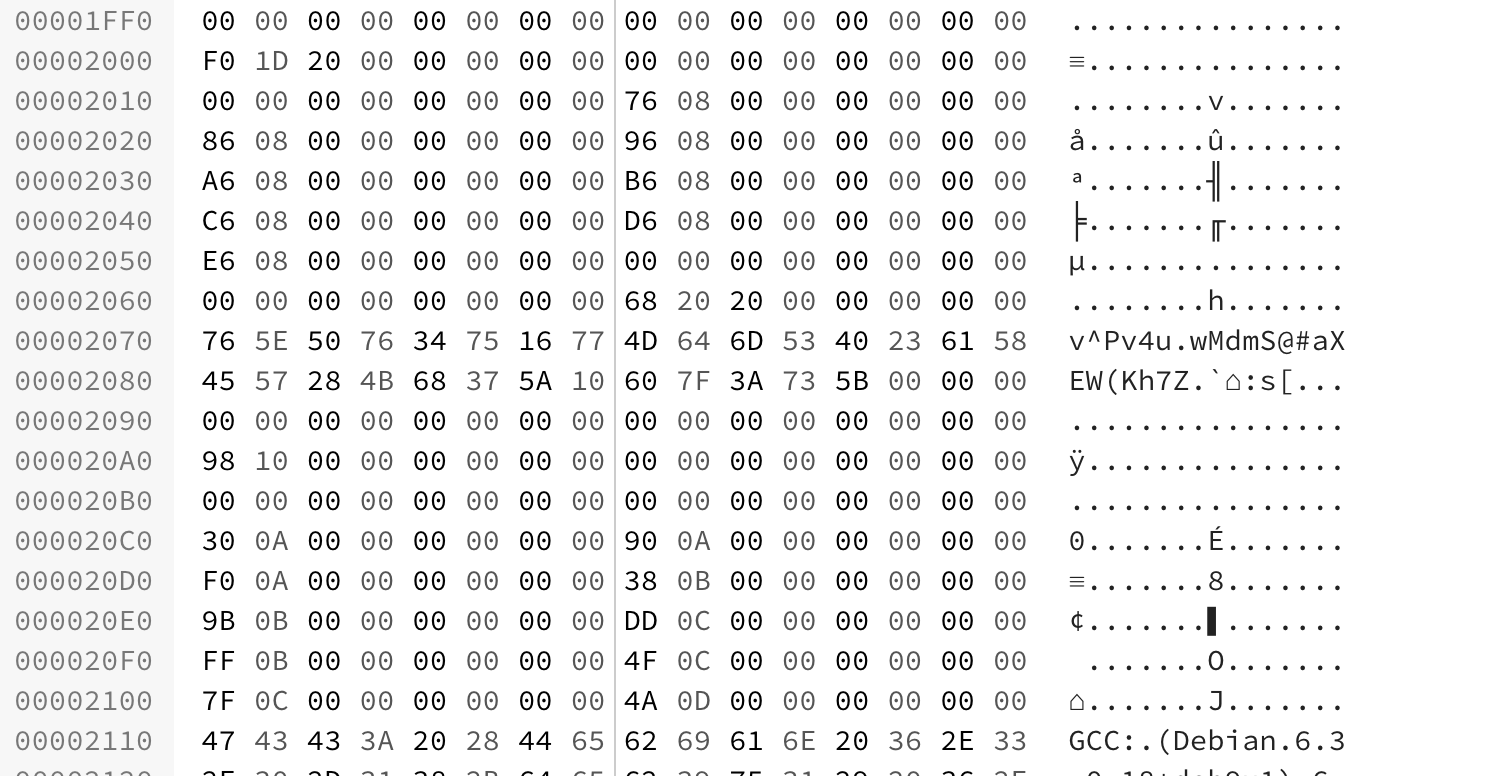Quals Sogeti Cyber E-scape 2019 - RE (500 pts).

### Challenge details

Event Challenge Category Points Solves
Quals Sogeti Cyber E-scape 2019 Bad VM Reverse 500 ?

### TL;DR

Look at the binary in a hex editor, spot the flag, XOR it with known plaintext. See that the key is decremented by 8 for each letter, bruteforce the modulo.

### Methology

I did this challenge without access to any reverse engineering tools. I only had an online hex editor and a python interpreter.

#### Look at the hex representationWith a bit of experience, a trained eye will see that some data is stored at offset 0x2070. This is encrypted data, probably something important, but what is more important in a CTF than the flag ?

So I extracted this data and assumed it was the flag.

#### Crytanalysis

The first thing that came to my mind after seeing this was : “This has to be a XOR”.

Because I knew the flag format, I performed a XOR with known plaintext on the first 4 bytes :

flag = "765E5076347516774D646D53402361584557284B68375A10607F3A735B".decode("hex")
known = "SCE{"

for i in range(4):
print(ord(flag[i])^ord(known[i]))


Which gave me this result :

37
29
21
13


Do you see what I see ? Each number is 8 less than the previous ! This means that the XOR key is decremented by 8 each time.

At this point I thought I was done. I scripted the decoding process :

flag = "765E5076347516774D646D53402361584557284B68375A10607F3A735B".decode("hex")

s = ""
k = 37
for e in flag:
s += chr(ord(e)^k)
k = (k-8)%256

print(s)


But the result was a bit unexpected :

SCE{1ã¨¹¸ÔõàÊ½ÆíJ/}"o>


The result is fine until the key gets negative. That’s when the modulo comes into play and that’s also the only thing we assumed to be 256, which it’s obviously not.

At this point a simple 254 possibilities bruteforce can recover the flag :

flag = "765E5076347516774D646D53402361584557284B68375A10607F3A735B".decode("hex")

for i in range(1, 255):
s = ""
k = 37
for e in flag:
s += chr(ord(e)^k)
k = (k-8)%i
print(i, s)


And there it was, with a modulo of 45 :

...
(42, 'SCE{1R\tBcDrY2hYfL;@k\x12G\x05mz\x1dlL')
(43, 'SCE{1]6o]lmp[0j[cI>En\x1e{\tqv;WG')
(44, 'SCE{1\\7n\\mlv]6l]lv1Za6\x7f\rur?Zz')
(45, 'SCE{1_4m_not_4n_is4_d3s1yn3r}')
(46, 'SCE{1^5l^onza:pQDp7\\g0w5}j7vp')
(47, 'SCE{1Y2kYhixc8rSF}\nQz=X9Af+zZ')
(48, 'SCE{1X3jXih~e>tU@z\rV}:_=Eb/~^')
...


And that’s how I solved this challenge in less than 10 minutes without even reversing it ;)

#### Flag

SCE{1_4m_not_4n_is4_d3s1yn3r}`

ENOENT